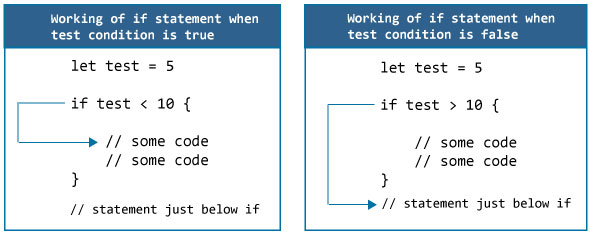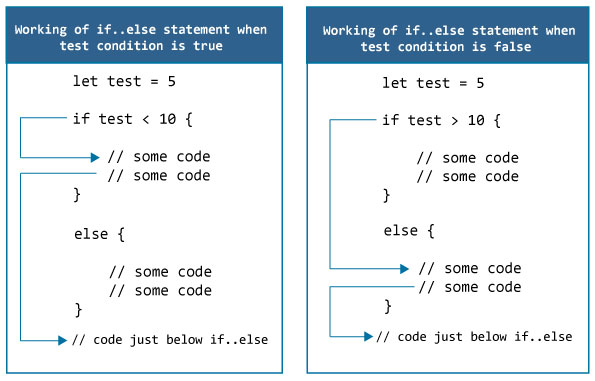Swift if, if...else Statement

# Swift if, if...else Statement

#### In this article, you will learn to use two conditional statements: if and if...else to control the flow of your program's execution.

In programming, you may want to perform different actions based upon the specified condition is `true` or `false` (which is known only during the run time). For such cases, control flow statements are used.

## Swift if (if-then) Statement

The syntax of if statement in Swift is:

```if expression {
// statements
}```
• Here `expression` is a boolean expression (returns either `true` or `false`).
• If the `expression` is evaluated to `true`, statements inside the code block of `if` is executed.
• If the `expression` is evaluated to `false`, statements inside the code block of `if` are skipped from execution.

### How if statement works?### Example 1: Swift if Statement

``````let number = 10
if number > 0 {
print("Number is positive.")
}
print("This statement is always executed.")
``````

When you run the program, the output will be:

```Number is positive.
This statement is always executed.```

In the above program, we have initialized constant number with value 10 and the test expression `number > 0` evaluates to true. Hence, the statement `print("Number is positive.")` inside the body of if statement is executed which outputs Number is positive. in the console..

Now, change the value of number to a negative integer. Let's say `-5`. The output in this case will be:

`This statement is always executed.`

When number is initialized with value -5, the test expression `number > 0` is evaluated to false. Hence, Swift compiler skips the execution of body of if statement.

## Swift if..else (if-else) Statement

The if statement executes a certain section of code if the test expression is evaluated to true. The if statement can have optional else statement. Code inside the body of else statement are executed if the test expression is false.

The syntax of if-else statement is:

```if expression {
// statements
} else {
// statements
}```

### How if..else statement works?### Example 2: Swift if else Statement

``````let number = 10
if number > 0 {
print("Number is positive.")
} else {
print("Number is not positive.")
}
print("This statement is always executed.")
``````

When you run the program, the output will be:

```Number is positive.
This statement is always executed```

In the above program, constant number is initialized with value 10 and the test expression `number > 0` evaluates to `true`. Hence,the statement `print("Number is positive.")` inside the body of if statement is executed.

This outputs Number is positive. in the console and the statements inside the body of else are skipped from execution.

Now, change the value of number to a negative number. Let's say -5. The output in this case will be:

```Number is not positive.
This statement is always executed.```

When number is -5, the test expression `number > 0` is evaluated to `false`. In this case, statements inside the body of else are executed, and statements inside the body of if are skipped from execution.

You can also replace if..else statement with ternary operator in Swift, which is kind of a shorthand notation of if...else statement.

## Swift if..else..if (if-else-if) Statement

In Swift, it's also possible to execute one block of code among many. For that, you can use if..else..if ladder as:

The syntax of if-else-if statement is:

```if expression1 {
// statements
}
else if expression2 {
// statements
}
else if expression3 {
// statements
}
.
.
else {
// statements
}```

The if statements are executed from the top towards the bottom. As soon as the test expression is true, codes inside the body of that if statement is executed. Then, the control of program jumps outside if-else-if ladder.

If all test expressions are `false`, code inside the body of else is executed

### Example 3: Swift if..else..if Statement

The following program checks whether number is positive, negative or 0.

``````let number = 0;
if number > 0 {
print("Number is positive.")
}
else if (number < 0) {
print("Number is negative.")
}
else {
print("Number is 0.")
}
``````

When you run the program, the output will be:

`Number is 0.`

In the above program, constant number is initialized with value 0. Since if statements are executed from top to bottom, it checks the expression `number > 0` which evaluates to `false`.

It then checks the next expression `number < 0` which also evaluates to false.

Hence, the statement `print("Number is 0.")` inside the body of else is executed which outputs Number is 0. in the console.

## Swift nested if..else Statement

It's possible to have if..else statements inside an if..else statement in Swift. It's called nested if...else statement.

You can also replace nested if..else statement with switch in Swift which is sometimes a simpler approach when dealing with several possible options.

The syntax of nested if-else statement is:

```if expression1 {
if expression2 {
// statements
} else {
// statements
}
}
else {
if expression3 {
// statements
} else {
// statements
}
}```

### Example 4: Nested if...else Statement

Here's a program to find largest of 3 numbers using nested if else statements.

``````let n1 = -1.0, n2 = 4.5, n3 = -5.3
if n1 >= n2 {
if n1 >= n3 {
print("Largest number is ", n1)
}
else {
print("Largest number is ", n3)
}
}
else {
if n2 >= n3 {
print("Largest number is ",  n2)
}
else {
print("Largest number is ",  n3)
}
}
``````

When you run the above program, the output will be:

`Largest number is 4.5`